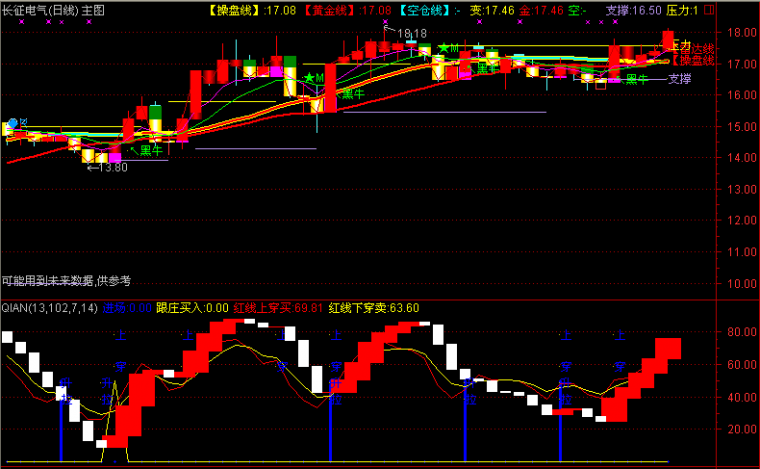﻿ 牛市有点用的选股-通达信公式 －程序化交易（CXH99.COM）

# 牛市有点用的选股[通达信公式]

V1赋值:10日内最低价的最低值
V2赋值:20日内最高价的最高值
LYF1赋值:((收盘价-V1)/(V2-V1))*((-100))的3日指数移动平均
VARG5赋值:((收盘价)/(5日前的收盘价)>=1.15)

V1:=LLV(LOW,10);
V2:=HHV(HIGH,20);
lyf1:=EMA(((CLOSE-V1)/(V2-V1))*((-100)),3);
VARg5:=((CLOSE)/(REF(CLOSE,5))>=1.15);

AND ((CLOSE)/(REF(CLOSE,1))>1.098) AND (REF(VOL,LOW)>REF(VOL,34))
AND (REF(VOL,LOW)>REF(VOL,1)) AND (LYF1>(-93))
AND (REF(VOL,LOW)>REF(VOL,8))),60,0);
cross(入市日 ,(58));(注：由于人数限制，QQ或微信请选择方便的一个联系我们就行，加好友时请简单备注下您的需求，否则无法通过。谢谢您！)

【字体： 】【打印文章】【查看评论

没有相关内容